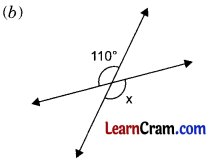# DAV Class 6 Maths Chapter 10 Brain Teasers Solutions

The DAV Maths Book Class 6 Solutions and DAV Class 6 Maths Chapter 10 Brain Teasers Solutions of Angles offer comprehensive answers to textbook questions.

## DAV Class 6 Maths Ch 10 Brain Teasers Solutions

Question 1.
A. Tick (✓) the correct answer.
What kind of angle do you get when you open any two adjacent fingers of your hand?
(i) right angle
(ii) obtuse angle
(iii) acute angle
(iv) straight angle
(ii) acute angle
When we open any two adjacent fingers of our hand, we get an acute angle between them.

(b) An angle measuring 240° is:
(i) obtuse angle
(ii) acute angle
(iii) supplementary angle
(iv) reflex angle
(iv) reflex angle
The angle which is more than 180° and less than 360° is called reflex angle.

(c) An supplement of an angle of 75° is
(i) 95°
(ii) 105°
(iii) 100°
(iv) 115°
(iii) 105°
When the sum of two angles is equal to 180°, then they are called supplementary angles to each other.

(d) The Complement of an angle of 55° is
(i) 45°
(ii) 125°
(iii) 35°
(iv) 135°
(iii) 35°
When the sum of two angles is equal to 90°, then they are called complementary angles to each other.

(e) How many right angles make one complete angle?
(i) 2
(ii) 3
(iii) 1
(iv) 4
(iv) 4
One complete angle has 360° i.e. 4 x 90°. This means that complete angle is made up of 4 right angles.(a) How many degrees are there in the angle made by the hour hand and the minute hand of a clock, when it is 9 o’clock?
90°.
When a clock strikes 9, its hour hand and minute hand stand vertical to each other. At 9 o’clock, hour hand is at 9 indicator while the minute hand is at 12 indicator and they form a right angle.

(b) Name of points in the exterior of ∠BCA but, in the interior of ∠ACD.Point S and point P.
Points S, P and R are in the exterior of ∠BCA but only points S and P are in the interior of ∠ACD. Point R is in the exterior of ∠ACD.

(c) State the kind of angle between the directions South-East and North.
Obsute angle.The kind of angle between South-East and North is 135° which is greater than 90° and smaller than 180°. So, it forms an obtuse angle.

(d) If the measure of one angle of a linear pair is of 85°, find the other angle. Find x is the given figure.
95°
If the two adjacent angles combine together to form an angle of 180°, then the adjacent angles is called a linear pairs.

(e) Find x is the given figure.320°
Here, x + 40° = 360°
⇒ x = 360° – 40° = 320°

Question 2.
How many degrees are there in
(a) $$\frac{2}{5}$$ right angle?
$$\frac{2}{5}$$ right angle(b) $$\frac{5}{6}$$ straight angle?
$$\frac{5}{6}$$ straight angle(c) $$\frac{2}{5}$$ complete angle?
$$\frac{2}{5}$$ complete angleQuestion 3.
Name the given angle in six different ways.∠a, ∠YXZ, ∠YXN, ∠MXN, ∠MXZ and ∠ZXY.

Question 4.
Complete the following using given figure:(a) Vertex of ∠1 is .
(b) Another name for ∠2 is .
(c) Another name for ∠1 + ∠2 is .
(d) Common arm of ∠1 and ∠2 is .
(e) ∠1 and ∠2 are angles.
(a) A
(d) ACQuestion 5.
While doing drill, Rohan was asked to about turn. After two minutes, he was again asked to about turn. How many degrees did Rohan turn in the activity?
In first turn, he moved through 180° and in second turn he again moved through 180°.
∴ Total angle moved in this activity = 180° + 180° = 360°.

Question 6.
(a) Can 2 acute angles be supplementary?
No

(b) Can 2 obtuse angles form a linear pair?
No

(c) Can linear pair be supplementary?
Yes

(d) Can 2 acute angles be complementry?
Yes

(e) Can vertically opposite angles be adjacent?
No

Question 7.
Look at the given figure and answer the following: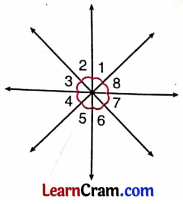(a) Sum of ∠1, ∠2, ∠3, ∠4, ∠5, ∠6, ∠7, ∠8.
(b) ∠3 = ____________
(Vertical angles).
(c) ∠5 = ____________
(Vertical angles).
(d) ∠4 and are adjacent angles.
(e) ∠1 + ∠2 + ∠3 + ∠5 + ∠6 + ∠7 + ∠8 = 320°.
What is the measure of ∠4?
(a) ∠1 + ∠2 + ∠3 + ∠4 + ∠5 + ∠6 + ∠7 + ∠8 = 360°
(b) ∠3 = ∠7
(c) ∠5 = ∠1
(d) ∠4 and ∠5; ∠4 and ∠3
(e) ∠4 = 360° – 320° = 40°Question 8.
Fill in the blanks:
(а) Sum of the angles forming a linear pair is always equal to ____________ degrees.
180°

(b) A reflex angle lies between ____________ and ____________.
180°; 360°

(c) An angle whose measure is equal to 360° is called a angle.
Complete

(d) The angle turned by the minute hand of a clock from the digit 2 to 8 is a angle and measures ____________ degrees.
straight, 180°

(e) The complement of 0.5° is ____________ degrees.
89.5°

### DAV Class 6 Maths Chapter 10 HOTS

Question 1.
Reena was standing facing North-West direction. She turns by 90° in anti-clockwise direction. Again she turns by 180° in anti-clockwise direction. Now she turns 45° in clockwise direction. Which direction is Reena facing now?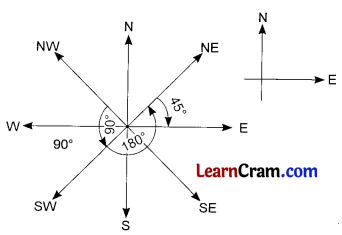Reena was standing facing North-West. She turns by 90° in anti-clockwise direction and she is facing South-West now. After that she turns by 180° in anti-clockwise direction and she is facing North-East direction. On turnning 45° in clockwise direction, she is facing East direction now.

Question 2.
Find the complement of the supplement of twice of an angle of 60°.
Twice of angle
60° = 2 x 60° = 120°
Thus, supplementary angle of 120° = 180° – 120° = 60°
Now, the complementary of 60° = 90° – 60° = 30°.

### DAV Class 6 Maths Chapter 10 Enrichment Questions

Question 1.
Observe the following letters of English alphabet.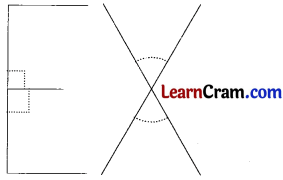We find a linear pair of angles in letter E and vertically opposite angles in letter X.
Now, make a list of letters in English alphabet which have linear pair and vertically opposite angles. Colour linear pair with green and vertically opposite angles with orange.
List of English Alphabet:All these English Alphabet form linear pairs. Only X forms vertically opposite angles.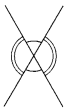Question 1.
Observe the given figure and answer the following: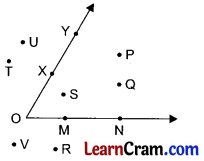(a) The points in the interior of the angle.
(b) The points in the exterior of the angle.
(c) Points in the angular region.
(a) The points in the interior of angle is P, Q and S.
(b) The points in the exterior of the angle are T, U, V and R.
(c) Angular points are Y, X, O, M, N, S, Q and P.

Question 2.
Name the following angles: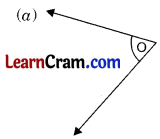∠O∠ABC∠PQR∠b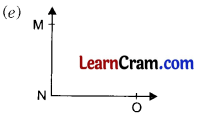∠MNO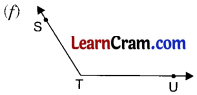∠STUQuestion 3.
Draw the following angles using pencil and scale only:
(a) Right angle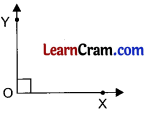(b) Straight angle(c) Obtuse angle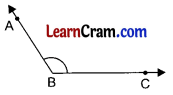(d) Reflex angle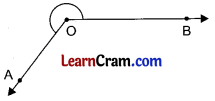(e) Acute angleQuestion 4.
Fill in the blanks:
(a) Acute angle is always less than ____________.
90°

(b ) Right angle is always equal to ____________.
90°

(c) Measure of straight angle is ____________.
180°

(d) Reflex angle is always greater than ____________.
180°

(e) Obtuse angle is always less than ____________.
180°

Question 5.
Write the kind of angles marked in the given figure: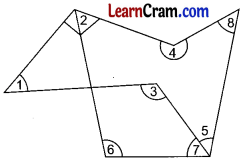∠1 is acute angle
∠2 is right angle
∠3 is obtuse angle
∠4 is reflex angle
∠5 is acute angle
∠6 is obtuse angle
∠7 is acute angle
∠8 is acute angle

Question 6.
Identify the complementary and supplementary angles from the following:
(a) 33°, 57°
(b) 120°, 60°
(c) 22$$\frac{1}{2}$$, 67$$\frac{1}{2}$$
(d) 0°, 90°
(e) 25°, 65°
(f) 89°, 1°
(a) 33° + 57° = 90°
they are complementary angles

(b) 120° + 60° = 180°
they are supplementary angles

(c) 22$$\frac{1}{2}$$ + 67$$\frac{1}{2}$$ = 90°
they are complementary angles

(d) 0° + 90° = 90°
they are complementary angles

(e) 25° + 65° = 90°
they are complementary angles

(f) 89° + 1° = 90°
they are complementary anglesQuestion 7.
Write the supplement of the given angles:
(a) 110°
180° – 110° = 70°

(b) 120°
180° – 120° = 60°

(c) 105°
180° – 105° = 75°

(d) 67$$\frac{1}{2}$$°
180° – 67$$\frac{1}{2}$$° = 112$$\frac{1}{2}$$°

(e) 38.5°
180° – 38.5° = 141.5°

(f) 25°
180° – 25° = 155°

(g) 65°
180° – 65° = 115°

(h) 0°
180° – 0° = 180°

(i) 1°
180° – 1° = 179°

(j) 63°
180° – 63° = 117°

Question 8.
Write true or false for the given statements:
(a) Reflex angle is always more than 90°.
False

(b) The sum of two angles of linear pair is 180°.
True

(c) The sum of supplementary angles is 180°.
True

(d) Vertically opposite angles are always equal.
True

(e) Two adjacent angles have a common arm.
True

(f) The measure of a complete angle is 360°.

(g) The complement of 55° is 135°.
False

(h) Angles of linear pair are not straight angles.
False

Question 9.
Observe the given figure and find the value of x.(3x + 5)° + (2x + 15)° = 180° (Linear pair)
⇒ 3x + 5° + 2x + 15° = 180°
⇒ 5x + 20° = 180°
⇒ 5x + 20° – 20° = 180° – 20°
⇒ 5x = 160°
⇒ 5x ÷ 5 = 160° ÷ 5
⇒ x = 32°Question 10.
Find the value of x in the following figures: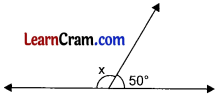x + 50° = 180° (Linear pair)
∴ x = 180° – 50° = 130°